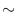Next: Error Handling ( error Up: Simple Data Types and Previous: Memory Management   Contents   Index

# Memory Allocator ( leda_allocator )

Definition

An instance A of the data type leda_allocator<T> is a memory allocator according to the C++standard. leda_allocator<T> is the standard compliant interface to the LEDA memory management.

#include < LEDA/system/allocator.h >

Types

Local types are size_type, difference_type, value_type, pointer, reference, const_pointer, and const_reference.

 template leda_allocator::rebind allows the construction of a derived allocator: leda_allocator::template rebind::other is the type leda_allocator.

Creation

 leda_allocator A introduces a variable A of type leda_allocator.

Operations

 pointer A.allocate(size_type n, const_pointer = 0) returns a pointer to a newly allocated memory range of size n * sizeof(T). void A.deallocate(pointer p, size_type n) deallocates a memory range of n * sizeof(T) starting at p. Precondition the memory range was obtained via allocate(n). pointer A.address(reference r) returns &r. const_pointer A.address(const_reference r) returns &r. void A.construct(pointer p, const_reference r) makes an inplace new new( (void*)p ) T(r). void A.destroy(pointer p) destroys the object referenced via p by calling p->T(). size_type A.max_size() the largest value n for which the call allocate(n,0) might succeed.

Implementation

Note that the above class template uses all kinds of modern compiler technology like member templates, partial specialization etc. It runs only on a subset of LEDA's general supported platforms like g++ > 2.95, SGI CC > 7.3.Next: Error Handling ( error Up: Simple Data Types and Previous: Memory Management   Contents   Index